﻿ 光滑深海立管涡激振动DES模拟
 舰船科学技术2016, Vol. 38Issue (3): 128-133PDF

1. 北京信息科技大学计算机学院, 北京 100101;
2. 北京信息科技大学网络文化与数字传播北京市重点实验室, 北京 100101

Numerical Simulation of Vortex Induced Vibration on Smooth Marine Riser Using DES Model
ZHU Min-ling1, 2, LV Xue-qiang2
1. School of Computer Science, Beijing Information Science and Technology University, Beijing 100101, China;
2. Beijing Key Laboratory of Internet Culture and Digital Dissemination Research, Beijing Information Science and Technology University, Beijing 100101, China
Abstract: DES (Detached Eddy Simulation) method was used to simulate vortex induced vibration of smooth marine riser. The height of first layer of the grid and Grid-independent solution and time step-independence solution is obtained. The lift coefficient, the drag coefficient, Strouhal number (St) and other results agree well with experimental data and other numerical results. The results show that, DES method based on SST k-ω turbulence model is credible and valid to simulate vortex induced vibration of smooth marine riser; the requirement of the first layer of the grid can be satisfied by 0.5Δy1.
Key words: DES    Marine riser    Vortex induced vibration    Grid    Time step
0 引言

1 数值模型建立 1.1 N-S 方程

 $\frac{{\partial {u_i}}}{{\partial {x_i}}} = 0,$ (1)
 $\frac{{\partial {u_i}}}{{\partial t}} + \frac{{\partial \left( {{u_i}{u_j}} \right)}}{{\partial {x_j}}} = - \frac{1}{\rho }\frac{{\partial p}}{{\partial {x_i}}} + \nu \frac{{{\partial ^2}{u_i}}}{{\partial {x_i}\partial {x_j}}},$ (2)

1.2 Menter k-ω SST 两方程湍流模式
 $\begin{array}{*{20}{l}} {\frac{{\partial \left( {\rho k} \right)}}{{\partial t}} + {u_i}\frac{{\partial \left( {\rho k} \right)}}{{\partial {x_i}}} = \;{P_k} - \frac{{\rho {k^{3/2}}}}{{{l_{k - \omega }}}} + \frac{\partial }{{\partial {x_i}}}\left[ {\left[ {{\mu _l} + \frac{{{\mu _l}}}{{{\sigma _k}}}} \right]\frac{{\partial k}}{{\partial {x_i}}}} \right],} \end{array}$ (3)
 $\begin{array}{*{20}{l}} {\frac{{\partial \left( {\rho \omega } \right)}}{{\partial t}} + {u_i}\frac{{\partial \left( {\rho \omega } \right)}}{{\partial {x_i}}} = {C_\omega }{P_\omega } - {\beta _\omega }\rho {\omega ^2} + \;\frac{\partial }{{\partial {x_i}}}\left[ {\left[ {{\mu _l} + \frac{{{\mu _l}}}{{{\sigma _k}}}} \right]\frac{{\partial k}}{{\partial {x_i}}}} \right] + \;2\rho \left( {1 - {F_1}} \right)\frac{1}{\omega }{\sigma _{{\omega ^2}}}\frac{{\partial k}}{{\partial {x_i}}}\frac{{\partial \omega }}{{\partial {x_i}}}.} \end{array}$ (4)

 ${\mu _l} = \min \left[{\frac{{\rho k}}{\omega },\frac{{{a_1}\rho k}}{{\Omega {F_2}}}} \right],$ (5)

 ${l_{k - \omega }} = \frac{{{k^{1/2}}}}{{{\beta _k}\omega }},$ (6)
F1F2为混合函数，PkPw为湍流生成项，具体定义根据参考文献给出。

1.3 DES 方法

DES 方法用长度尺度替代 k- SST 中长度尺度，从而使得计算区域在附面层使用 k- SST 模型，在主流分离区域使用大涡模拟模型。

 $l = min\left[{{l_{k - \omega }},{C_{{\text{DES}}}}\Delta } \right],$ (7)

2 研究对象与数值方法 2.1 计算域及其离散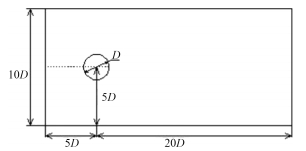图 1 计算域示意图 Fig. 1 Fluid computational domain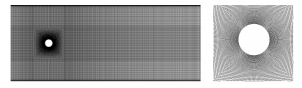图 2 离散网格及放大图 Fig. 2 Mesh and its local magnification
2.2 数值方法与边界条件

3 结果及分析 3.1 网格第一层高度对结果影响

 ${y^ + } = 0.172\frac{{\Delta y}}{D}{\text{ }}R{e^{0.9}},$ (8)

NASA 粘性网格厚度计算器也可估算网格第一层高度。NASA 粘性网格厚度计算器是基于空气介质在平板湍流中按照 Sutherland’s law 来计算空气粘性厚度，估算Δy。本文中，按 NASA 粘性网格厚度计算器估算得到的第一层网格高度称为Δy2。计算得到Δy1 > Δy2表 1 不同网格第 1 层高度 Re = 200 计算结果 Tab.1 Calculated results of different Δy when Re = 200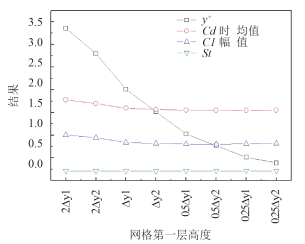图 3 Re = 200 计算结果随网格第一层高度变化规律 Fig. 3 Calculated results of different Δy when Re = 200表 2 本文部分网格无关解结果与文献数据的比较 Tab.2 Comparison between calculated results of different meshes with the references
3.2 网格数量对结果影响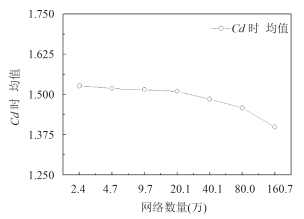图 4 阻力系数时均值随网格数变化规律 Fig. 4 Drag coefficient of different meshes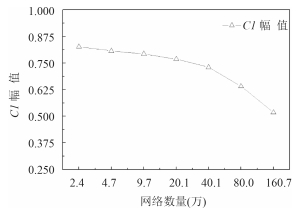图 5 升力系数幅值随网格数变化规律 Fig. 5 lift coefficient of different meshes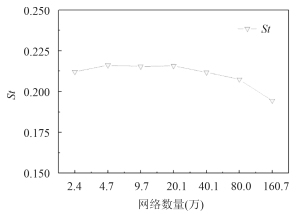图 6 斯特罗哈尔数随网格数变化规律 Fig. 6 Strouhal number of different meshes
3.3 时间步长对结果影响表 3 两组网格 Re = 200 不同时间步长计算结果 Tab.3 Calculated results of different time steps when Re = 200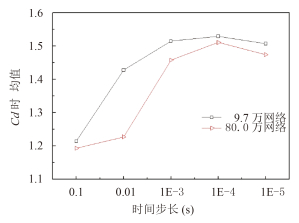图 7 阻力系数时均值随时间步长变化规律 Fig. 7 Drag coefficient of different time steps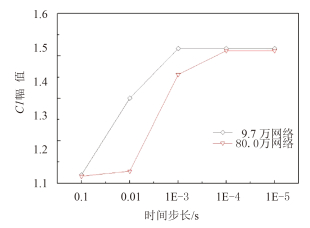图 8 升力系数幅值随时间步长变化规律 Fig. 8 Lift coefficient of different time steps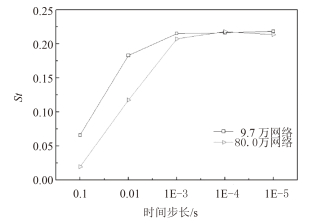图 9 斯特罗哈尔数随时间步长变化规律 Fig. 9 Strouhal number of different time steps

3.4 Re = 200 结果表 4 Re = 200 计算结果 Tab.4 Calculated results when Re = 200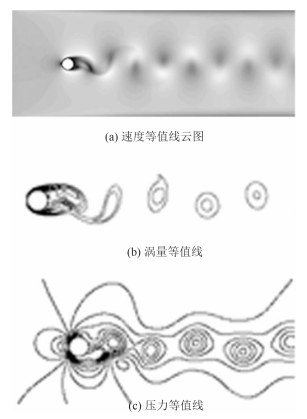图 10 Re = 200 瞬时流场等值线云图 Fig. 10 Transient contours when Re = 200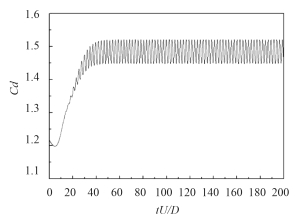图 11 Re = 200 阻力时程曲线 Fig. 11 Drag coefficient vs time when Re = 200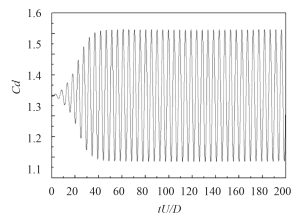图 12 Re = 200 升力时程曲线 Fig. 12 Lift coefficient vs time when Re = 200
4 结语

1）基于 SST k-ω 湍流模型的 DES 方法模拟低雷诺数圆柱绕流涡激振动结果合理；

2）随着网格第一层高度Δy 的减小，y+降低。按 0.5Δy1 确定 DES 方法的第一层网格高度可得到满足要求的；

3）增加网格数量可使计算结果更接近实验结果，但网格数量到一定程度后再增加对结果改善不明显。本文中 80.0 万网格结果最优；

4）减小时间步长可提高计算精度，需针对不同对象进行时间步长无关解研究。

  胡伟. 高耸结构绕流与流固耦合的数值模拟[D]. 西安:西安建筑科技大学, 2010. HU Wei. Numerical simulation of wind pass high-rise structure and fluid-solid coupling[D]. Xi'an:Xi'an University of Architecture and Technology, 2010.  曹淑刚, 黄维平, 顾恩凯. 考虑流固耦合的弹性圆柱体涡激振动研究[J]. 振动与冲击, 2015, 34(1):58-62. CAO Shu-gang, HUANG Wei-ping, GU En-kai. Study on vortex-induced vibration of an elastic cylinder considering fluid-structure interaction[J]. Journal of vibration and shock, 2015, 34(1):58-62.  蒋仁杰. 圆柱绕流场涡致柱体振动的研究[D]. 杭州:浙江大学, 2013. JIANG Ren-jie. Research on vortex-induced vibrations in the flow around circular cylinders[D]. Hangzhou:Zhejiang University, 2013.  丁林, 张力, 杨仲卿. 高雷诺数时分隔板对圆柱涡致振动的影响[J]. 机械工程学报, 2013, 49(14):133-139. DING Lin, ZHANG Li, YANG Zhong-qing. Effect of splitter plate on vortex-induced vibration of circular cylinder at high Reynolds number[J]. Journal of mechanical engineering, 2013, 49(14):133-139.  李骏, 李威. 基于SST k-ω湍流模型的二维圆柱涡激振动数值仿真计算[J]. 舰船科学技术, 2015, 37(2):30-34. LI Jun, LI Wei. Numerical simulation of vortex-induced vibration of a two-dimensional circular cylinder based on the SST k-ω turbulent model[J]. Ship science and technology, 2015, 37(2):30-34.  STRELETS M. Detached eddy simulation of massively separated flows[R]. AIAA-01-0879. San Antonio, Texas:American Institute of Aeronautics & Astronautics, 2001.  MENTER R. Zonal Two equation k-turbulence models for aerodynamics flows[R]. AIAA-93-2906. Orlando, FL:American Institute of Aeronautics & Astronautics, 1993.  顾春伟, 陈美兰, 李雪松, 等. DES模型在压气机叶栅中的应用研究[J]. 工程热物理学报, 2008, 29(12):2007-2010. GU Chun-wei, CHEN Mei-lan, LI Xue-song, et al. Application of DES model in the compressor cascade flow[J]. Journal of engineering thermophysics, 2008, 29(12):2007-2010.  时忠民, 刘名名, 郭晓玲. 计算域对圆柱绕流数值模拟结果的影响[J]. 中国水运, 2013, 13(7):83-88. SHI Zhong-min, LIU Ming-ming, GUO Xiao-ling. Effect of flow field on flow around circular cylinder[J]. China water transport, 2013, 13(7):83-88. (未链接到本条文献英文信息)  SPALART P R. Young-person's guide to detached-eddy simulation grids[R]. NASA/CR-2001-211032. Washington:Boeing Commercial Airplanes, 2001  常书平, 王永生, 庞之洋. 用基于SST模型的DES方法数值模拟圆柱绕流[J]. 舰船科学技术, 2009, 31(2):30-33. CHANG Shu-ping, WANG Yong-sheng, PANG Zhi-yang. Numerical simulation of flow around circular cylinder using SST DES model[J]. Ship science and technology, 2009, 31(2):30-33.  NASA. Viscous grid spacing calculator[EB/OL]. 1997. http://geolab.larc.nasa.gov/APPS/YPlus/.  SUTHERLAND W. The viscosity of gases and molecular force[J]. Philosophical magazine, 1893, 36(223):507-531  BRAZA M, CHASSAING P, MINH H H. Numerical study and physical analysis of the pressure and velocity fields in the near wake of a circular cylinder[J]. Journal of fluid mechanics, 1986, 165:79-130  魏志理, 孙德军, 尹协远. 圆柱尾迹流场中横向振荡翼型绕流的数值模拟[J]. 水动力学研究与进展(A辑), 2006, 21(3):299-308. WEI Zhi-li, SUN De-jun, YIN Xie-yuan. A numerical simulation of flow around a transversely oscillating hydrofoil in the wake of a circular cylinder[J]. Journal of Hydrodynamics, 2006, 21(3):299-308.